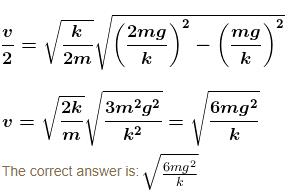Courses

# Work, Power And Energy MCQ Level - 2

## 10 Questions MCQ Test Topic wise Tests for IIT JAM Physics | Work, Power And Energy MCQ Level - 2

Description
This mock test of Work, Power And Energy MCQ Level - 2 for IIT JAM helps you for every IIT JAM entrance exam. This contains 10 Multiple Choice Questions for IIT JAM Work, Power And Energy MCQ Level - 2 (mcq) to study with solutions a complete question bank. The solved questions answers in this Work, Power And Energy MCQ Level - 2 quiz give you a good mix of easy questions and tough questions. IIT JAM students definitely take this Work, Power And Energy MCQ Level - 2 exercise for a better result in the exam. You can find other Work, Power And Energy MCQ Level - 2 extra questions, long questions & short questions for IIT JAM on EduRev as well by searching above.
QUESTION: 1

### The blocks A and B shown in the figure have masses MA = 5kg and MB = 4 kg. The system is released from rest. The speed of B after A has traveled a distance 1 m along the incline is :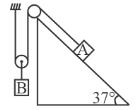Solution:

If A moves down the incline by 1 metreB shall move up by 1/2 metre. If the speed of B is v then the speed of A will be 2v.
Gain in K.E. = loss in P.E.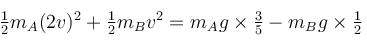Solving, we get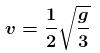The correct answer is: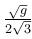QUESTION: 2

### In the figure the variation of components of acceleration of a particle of mass 1kg is shown w.r.t. time. The initial velocity of the particle is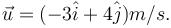The total work done by the resultant force on the particle in time interval from t = 0 to t = 4 second is :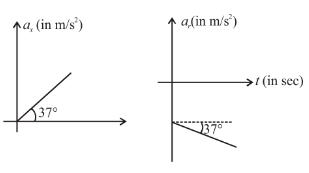Solution: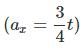and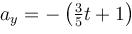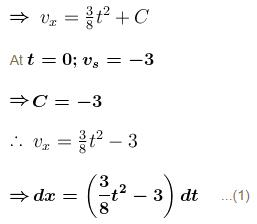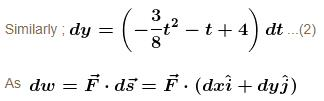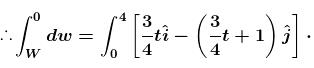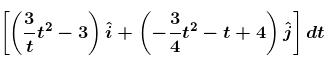∴ W = 10 J

QUESTION: 3

### A block of mass m is being pulled up the rough incline by the agent delivering constant power P. The coefficient of friction between the block and the incline is µ. The maximum speed of the block during the courses of ascent is :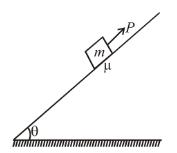Solution:

Let at any time the speed of the block along the incline upwards be v.
Then from Newton’s second law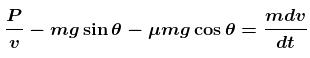the speed is maximum when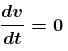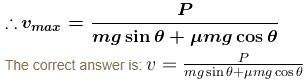QUESTION: 4

The potential energy (in SI units) of a particle of mass 2kg in a conservative fields U = 6x – 8y. If the  initial velocity of the particle is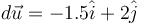then the total distance traveled by the particle in first two seconds is :

Solution: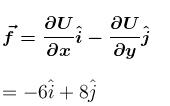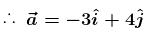has same direction as that of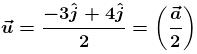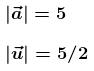Since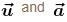are in same direction, particle will move along a straight line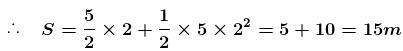QUESTION: 5

The potential energy of a particle of mass m free to move along x-axis is given by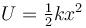for x < 0 and U = 0 for  x ≥ 0 (x denotes the x-coordinate of the particle and k is a positive constant). If the total mechanical energy of the particle is E, then its speed at x = -(2E/k)1/2

Solution:

From conservation of energy
K.E. + P.E. = E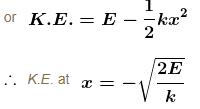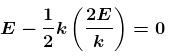∴
The speed of particle at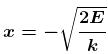is zero.

QUESTION: 6

Work done by static friction on an object :

Solution: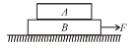Consider the blocks shown in the figure to be moving together due to friction between them. The free body diagrams of both the blocks are shown below.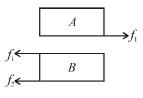Work done by static friction on A is positive and on B is negative.
The correct answer is: may be positive

QUESTION: 7

A man places a chain (of mass m and length l) on a table slowly. Initially the lower end of the chain just touches the table. The man drops the chain. When half of the chain is in vertical position, the potential energy of chain is :

Solution:

The work done by man is negative of magnitude of decrease in potential energy of chain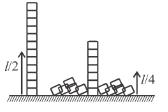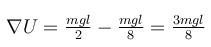The correct answer is: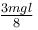QUESTION: 8

A collar B of mass 2kg is constrained to move along a horizontal smooth and fixed circular track of radius 5m. The spring lying in the plane of the circular track and having spring constant 200N/m is undeformed when the collar is at A. If the collar starts from rest at B the normal reaction exerted by the track on the collar when it passes through A is :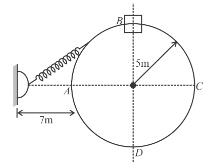Solution:

Initial extension will be equal to 6 m.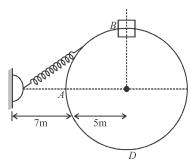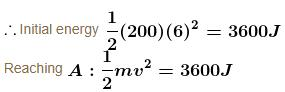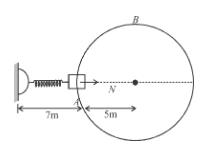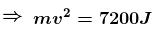From F.B.D. at A :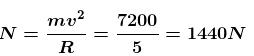QUESTION: 9

The spring block system lies on a smooth horizontal surface. The free end of the spring is being pulled towards right with constant speed v0 = 2m/s. At t = 0 sec, the spring of constant k = 100 N/cm in unstretched and the block has a speed 1m/s to left. The maximum extension of the spring is :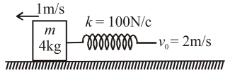Solution:

In the frame (inertial w.r.t. earth) of free end of spring, the initial velocity of block is 3 m/s to left and the spring unstretched.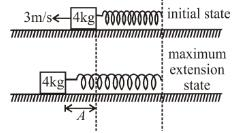Applying conservation of energy between initial and maximum extension state.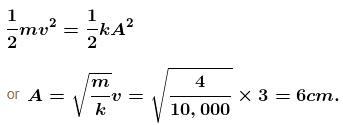QUESTION: 10

Block A is hanging from a vertical spring and is at rest. Block B strikes the block A with velocity v and sticks to it. Then the value of v for which the spring just attains natural length is :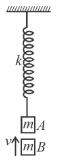Solution:

The initial extension in spring is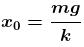Just after collision of B with A the speed of combined mass is v/2.

For the spring to just attain natural length the combined mass must rise up by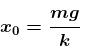(see fig.) and comes to rest.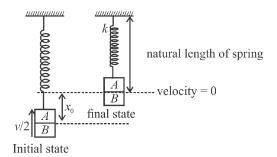Applying conservation of energy between initial and final states.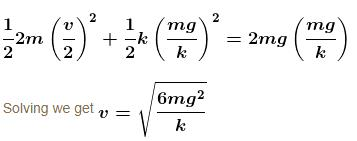Alternate solution by SHM